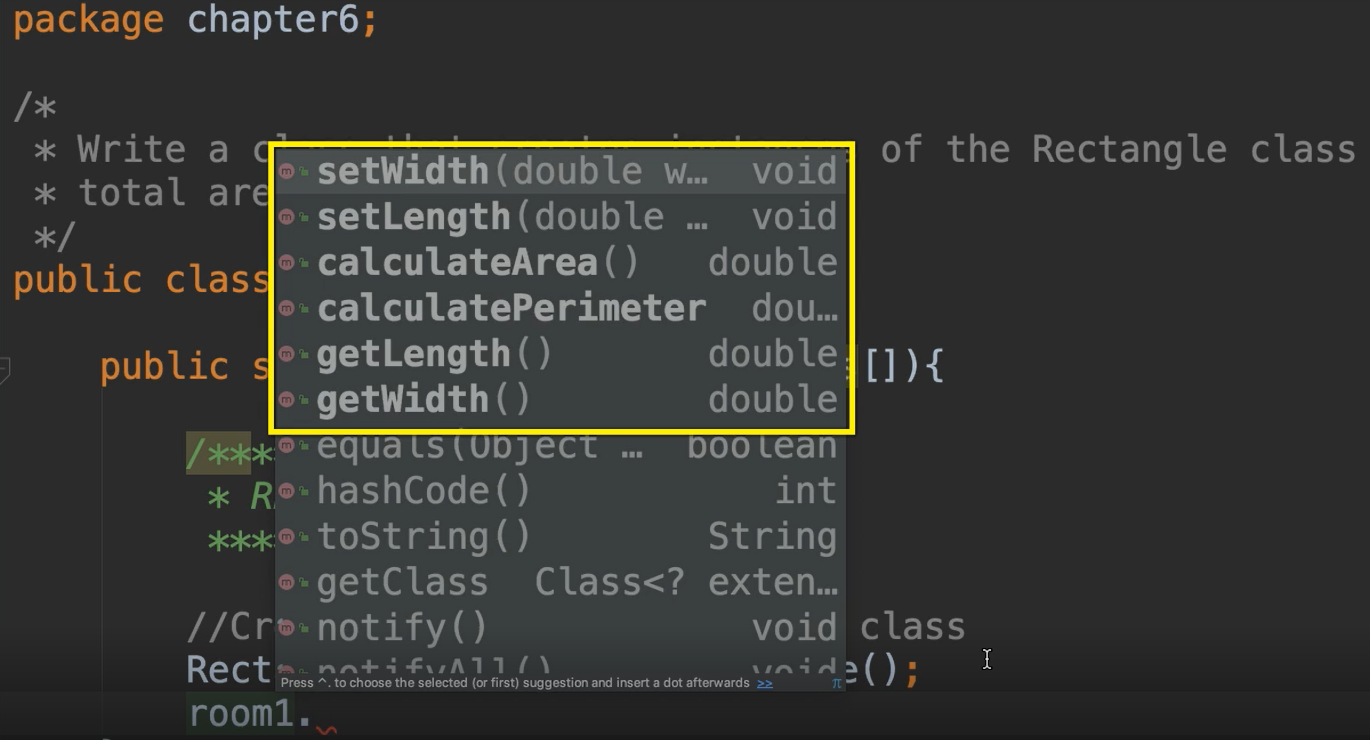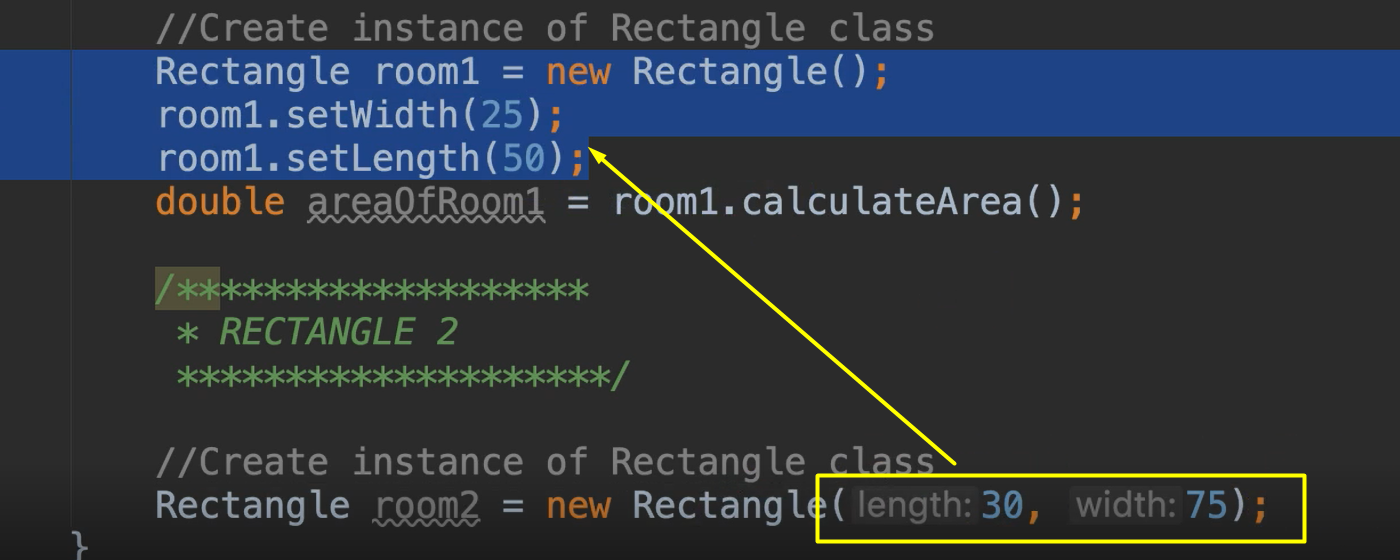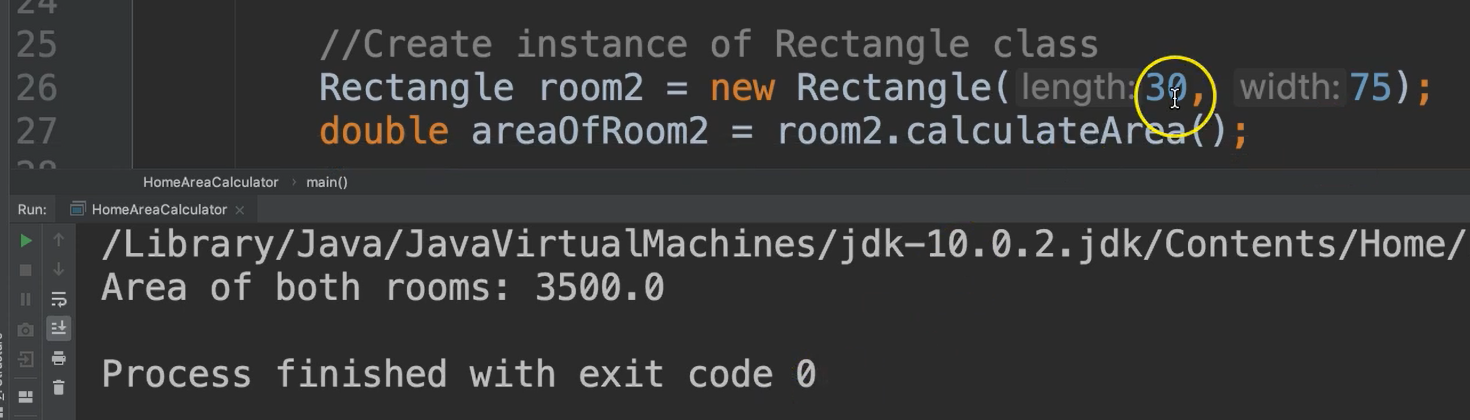Transcripted Summary

In the last section, we created a class for `Rectangle`.

Now we're going to create a new class, `HomeAreaCalculator`.

``````package chapter6;

/*
* Write a class that creates instances of the `Rectangle` class to find the
* total area of two rooms in a house.
*/
public class HomeAreaCalculator {

public static void main(String args[]){

}
}
``````

In this class, we're going to create two rooms by instantiating the `Rectangle object`.

In this class we need to create instances of the `Rectangle` class to find the total area of two rooms in a house.

So, let's go ahead and make the first rectangle.

We'll just put some comments here so we can keep track of what we're doing.

``````/*******************
* RECTANGLE 1
********************/
``````

Let's create an instance of the `Rectangle` class.

What we're doing here is creating a new object. We're going to give it a data type, but the data types are changing now. It's not just limited to the primitive data types that come with Java, we can give them class types as well.

So let’s declare this as type `Rectangle` — this is referencing this `Rectangle` class (from our last section).

So, we say we have a type of object, it's type is `Rectangle` and we'll name this object `room1`.

This is where we create the instantiation. To create an instantiation or an instance of an object we use the key word `new`, followed by the constructor of the class we are creating object of.

``````Rectangle room1 = new Rectangle();
``````

Let's examine this statement, so we have `Rectangle` which is the data type for this object, `room1`.

We are calling the constructor of `Rectangle`, but which constructor? Because, remember, we have two constructors. We are calling whichever one matches this parameter list.

So, this is the default one, meaning we are calling this one [from the previous section]:And it's going to set `length` to 0 and it's going to set `width` to 0.

Now let's say we want to give some values to the length and the width.

We can say `room1` (this is the object), and access the object’s methods using the dot operator (`.`).Notice here now we have a list of all of the methods that are available to us from this `Rectangle` object.

We're going to call `setWidth()`, and we can give this anything, let's say 25. We'll say now `setLength()` and we'll give this 50.

So, we've created a new object, `room1` of type `Rectangle` and we've set the width and we've set the length. At this point we can do any calculations we wanted to do.

Let's say that we want to calculate the area, since that's what our program asks us to do.

``````//Create instance of Rectangle class
Rectangle room1 = new Rectangle();
room1.setWidth(25);
room1.setLength(50);
double areaOfRoom1 = room1.calculateArea();
``````

Now let's create another rectangle.

We'll call this one rectangle two.

``````/*******************
* RECTANGLE 2
********************/
``````

We're going to create another instance of the `Rectangle` class.

``````//Create instance of Rectangle class
Rectangle room2 = new Rectangle()
``````

This time, instead of using the default constructor, I'm going to show you where we're going to use the other constructor which took 2 arguments, `length` followed by `width`.

So, we'll give these some different values. We'll say 30 for `length` and 75 for `width`.So, this line has essentially done the same thing that these three lines have done by using a different constructor. And note that this is for a different instance of the `Rectangle` class.

So, now we can get the area for `room2` by saying:

``````//Create instance of Rectangle class
Rectangle room2 = new Rectangle(30, 75);
double areaOfRoom2 = room2.calculateArea();
``````

Now that we have the 2 areas, we can get the `totalArea` by simply adding them together.

``````//Create instance of Rectangle class
Rectangle room2 = new Rectangle(30, 75);
double areaOfRoom2 = room2.calculateArea();
double totalArea = areaOfRoom1 + areaOfRoom2;
``````

Then we can print it out.

### # HomeAreaCalculator.java

``````package chapter6;

/*
* Write a class that creates instances of the `Rectangle` class to find the
* total area of two rooms in a house.
*/
public class HomeAreaCalculator {

public static void main(String args[]){

/*******************
* RECTANGLE 1
********************/

//Create instance of `Rectangle` class
Rectangle room1 = new Rectangle();
room1.setWidth(25);
room1.setLength(50);
double areaOfRoom1 = room1.calculateArea();

/*******************
* RECTANGLE 2
********************/

//Create instance of `Rectangle` class
Rectangle room2 = new Rectangle(30, 75);
double areaOfRoom2 = room2.calculateArea();

double totalArea = areaOfRoom1 + areaOfRoom2;

System.out.println("Area of both rooms: " + totalArea);
}
}
``````

As you can see, we've used this `Rectangle` class in a different way than it may have been originally designed for.

This `Rectangle` class does not care how we use it. It's basically used as a blueprint for any type of rectangle. And what we've chosen to use it for is to represent a room within a house, which happens to also be a type of rectangle. You can use this class in any way you want from other classes to represent the object in the way that you want it to.

So, this `Rectangle`, this class [that we created previously], is essentially a definition.** **It's a blueprint that you can use however you want to.

Then in here when we created an instance, this is a specific instance of this general class. So now we have a specific instance that we are going to use to do whatever we want to do.

So, let's just run this.We see that the area of both rooms is 3500.